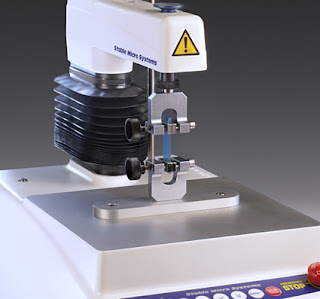# Texture Analysis Professionals Blog

How to measure and analyse the texture of food, cosmetics, pharmaceuticals and adhesives.

## Tuesday, 25 September 2018

### Tensile Testing using a Texture Analyser – Calculating True Stress and True StrainThe calculation of parameters from the stress-strain graph of a tensile test has already been covered in a previous blog post.

The calculations considered were those most often used when referring to stress and strain, and to give them their full name they would be called “engineering stress” and “engineering strain”. It is usually safe to assume that every time stress and strain are mentioned in the literature, this refers to the engineering values.

However, as the load on a sample increases, the cross-section over which the force is applied changes (it gets thinner). If the engineering stress is used (taking into account the initial area), the stress is underestimated. True stress solves this issue by using the instantaneous area over the course of application of load so that as the cross-section changes, the value of stress is calculated using the new cross-sectional area.
Additionally, whereas engineering strain is the amount that a material deforms per unit length, true strain is the natural log of the current length over the original length.